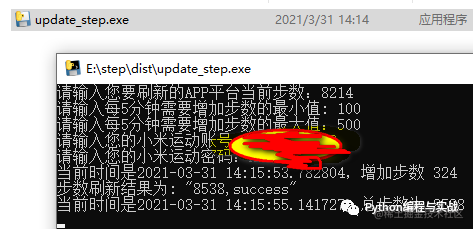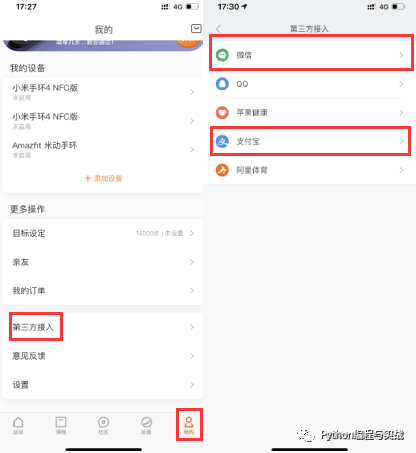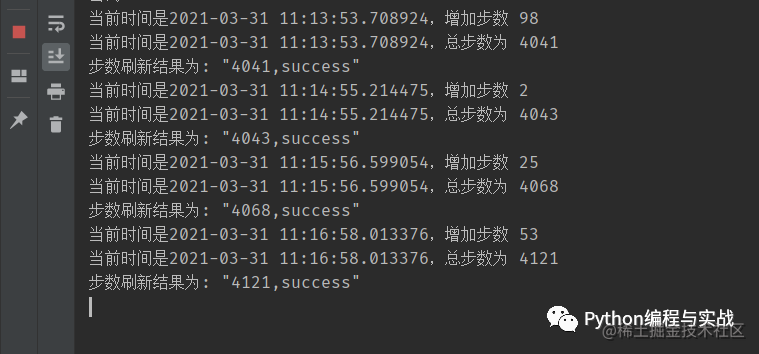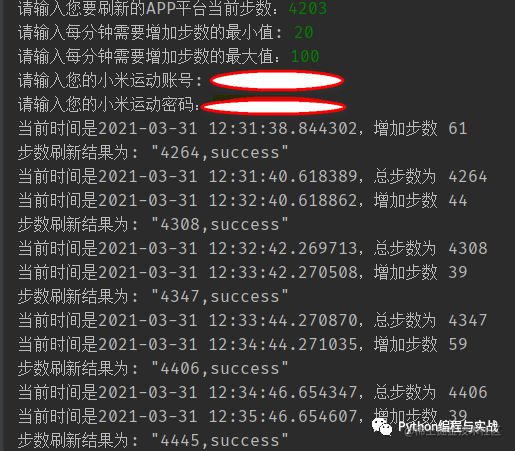# 使用 Python 修改微信/QQ/支付宝运动步数SO!  我已帮你打包成软件，在电脑上运行即可1. 下载安装程序
2. 运行 Python 脚本或运行程序

### 安装### Python 修改步数

``````if 8 <= hour <= 22:
random_step = random.randint(a, b)
print(f"当前时间是{datetime.now()}，增加步数 {random_step}")
step += random_step
if step >= 98800:
print("您的步数已达到上限，不要太贪心噢~~")
break